Gearing towards digital Education    0716 858 334

# Acids, Bases and Salts - Form 4 Chemistry

Change Topic

### Guest Account

Attempt Form Four Chemistry Questions
These notes are free for all students and teachers.

Create an account / login to help track your progress and unlock more features including additional notes, rapid quizzes, revision questions, virtual library, and the new Esoma Classroom

# Acids, Bases and Salts - Chemistry Form 4 Notes

Last Updated: 19-12-2021 | Esoma-KE

## Acids and Bases

- At a school laboratory:
- An acid may be defined as a substance that turn litmus red.
- A base may be defined as a substance that turn litmus blue.
- Litmus is a lichen found mainly in West Africa.
- It changes its colour depending on whether the solution it is in, is basic/alkaline or acidic.
- It is thus able to identify/show whether.

1. An acid is a substance that dissolves in water to form H+/H3O+ as the only positive ion/cation.
- This is called the Arrhenius definition of an acid.
- From this definition, an acid dissociate/ionize in water releasing H+ thus:
HCl(aq) → H+ (aq) + Cl- (aq)
HNO3(aq) → H+ (aq) + NO3-(aq)
CH3COOH(aq) → H+ (aq) + CH3COO-(aq)
H2SO4(aq) → 2H+ (aq) + SO42-(aq)
H2CO3(aq) → 2H+ (aq) + CO32-(aq)
H3PO4(aq) → 3H+ (aq) + PO43-(aq)

2. A base is a substance which dissolves in water to form OH- as the only negatively charged ion/anion.
- This is called Arrhenius definition of a base. - From this definition, a base dissociate/ionize in water releasing OH- thus:
KOH(aq) → K+(aq) + OH-(aq)
NaOH(aq) → Na+(aq) + OH-(aq)
NH4OH(aq) → NH4+(aq) + OH-(aq)
Ca(OH)2(aq) → Ca2+(aq) + 2OH-(aq)
Mg(OH)2(aq) → Mg2+(aq) + 2OH-(aq)

3. An acid is a proton donor.
- A base is a proton acceptor. - This is called Bronsted-Lowry definition of acids and bases. - From this definition, an acid donates H+. - H+ has no electrons and neutrons .It contains only a proton.

### Examples

I. From the equation:
HCl(aq) + H2O(l) === H3O+(aq) + Cl- (aq)
(a)
(i) For the forward reaction from left to right, H2O gains a proton to form H3O+ and thus H2O is a proton acceptor. It is a Bronsted-Lowry base.
(ii) For the backward reaction from right to left, H3O+ donates a proton to form H2O and thus H3O+ is an ‘opposite’ proton donor. It is a Bronsted-Lowry conjugate acid.

(b)
(i) For the forward reaction from left to right, HCl donates a proton to form Cl- and thus HCl is a proton donor. It is a Bronsted-Lowry acid.
(ii) For the backward reaction from right to left, Cl- gains a proton to form HCl and thus Cl- is an ‘opposite’ proton acceptor.It is a Bronsted-Lowry conjugate base.
Every base /acid from Bronsted-Lowry definition thus must have a conjugate product/reactant.

II. From the equation:
HCl(aq) + NH3(aq) === NH3+(aq) + Cl- (aq)
(a)
(i) For the forward reaction from left to right, NH3 gains a proton to form NH4+ and thus NH3 is a proton acceptor.
It is a Bronsted-Lowry base.
(ii) For the backward reaction from right to left, NH4+ donates a proton to form NH3 and thus NH4+ is an ‘opposite’ proton donor.
It is a Bronsted-Lowry conjugate acid.
(b)
(i) For the forward reaction from left to right, HCl donates a proton to form Cl- and thus HCl is a proton donor. It is a Bronsted-Lowry acid.
(ii) For the backward reaction from right to left, Cl- gains a proton to form HCl and thus Cl- is an ‘opposite’ proton acceptor. It is a Bronsted-Lowry conjugate base.

4. Acids and bases show acidic and alkaline properties/characteristics only in water but not in other solvents e.g.
(a)Hydrogen chloride gas dissolves in water to form hydrochloric acid Hydrochloric acid dissociates/ionizes in water to free H+(aq)/H3O+(aq) ions. The free H3O+(aq) / H+(aq) ions are responsible for:
1. Turning blue litmus paper/solution red.
2. Show pH value 1/2/3/4/5/6.
3. Are good electrolytes/conductors of electricity/undergo electrolysis.
4. React with metals to produce / evolve hydrogen gas and a salt. i.e.

#### Ionically:

For a monovalent metal: 2M(s) + 2H+(aq) → 2M+(aq) + H2(g)
For a divalent metal: M(s) + 2H+(aq) → M2+(aq) + H2(g)
For a trivalent metal: 2M(s) + 6H+(aq) → 2M3+(aq) + 3H2(g)
Examples:
For a monovalent metal: 2Na(s) + 2H+(aq) → 2Na+(aq) + H2(g)
For a divalent metal: Ca(s) + 2H+(aq) → Ca2+(aq) + H2(g)
For a trivalent metal: 2Al(s) + 6H+(aq) → 2Al3+(aq) + 3H2(g)

5. React with metal carbonates and hydrogen carbonates to produce /evolve carbon(IV)oxide gas ,water and a salt. i.e.

#### Ionically:

For a monovalent metal:
M2CO3(s)+ 2H+(aq) → 2M+(aq) + H2O (l)+ CO2(g)
MHCO3(s)+ H+(aq) → M+(aq) + H2O (l)+ CO2(g)
For a divalent metal: MCO3(s)+ 2H+(aq) → M2+(aq) + H2O (l)+ CO2(g)
M(HCO3)2(aq)+2H+(aq) →M2+(aq)+2H2O(l)+2CO2(g)
Examples:
For a monovalent metal: K2CO3(s)+ 2H+(aq) → 2K+(aq) + H2O (l)+ CO2(g)
NH4HCO3(s)+ H+(aq) → NH4+(aq) + H2O (l)+ CO2(g)
For a divalent metal: ZnCO3(s)+ 2H+(aq) → Zn2+(aq) + H2O (l)+ CO2(g)
Mg(HCO3) 2(aq)+2H+(aq) →Mg2+(aq)+2H2O(l)+2CO2(g)
(vi)neutralize metal oxides/hydroxides to salt and water only. i.e.

#### Ionically:

For a monovalent metal: M2O(s) + 2H+(aq) → 2M+(aq) + H2O (l)
MOH(aq) + H+(aq) → M+(aq) + H2O (l)
For a divalent metal: MO(s) + 2H+(aq) → M2+(aq) + H2O (l)
M(OH) 2(s) + 2H+(aq) → M2+(aq) + 2H2O(l)
For a trivalent metal: M2O3(s) + 6H+(aq) → 2M3+(aq) + 3H2O (l)
M(OH) 3(s) + 3H+(aq) → M3+(aq) + 3H2O(l)
Examples:
For a monovalent metal: K2O(s) + 2H+(aq) → 2K+(aq) + H2O (l)
NH4OH(aq) + H+(aq) → NH4+(aq) + H2O (l)
For a divalent metal: ZnO (s) + 2H+(aq) → Zn2+(aq) + H2O (l)
Pb(OH) 2(s) + 2H+(aq) → Pb2+(aq) + 2H2O(l)
(b) Hydrogen chloride gas dissolves in methylbenzene /benzene but does not dissociate /ionize into free ions.
It exists in molecular state showing none of the above properties.
(c) Ammonia gas dissolves in water to form aqueous ammonia which dissociate/ionize to free NH4+ (aq) and OH-(aq) ions.
This dissociation/ionization makes aqueous ammonia to:
(i) turn litmus paper/solution blue.
(ii) have pH 8/9/10/11.
(iii) be a good electrical conductor.
(iv) react with acids to form ammonium salt and water only.
NH4OH(aq) + HCl(aq) → NH4Cl(aq) + H2O(l)
(d) Ammonia gas dissolves in methylbenzene/benzene /kerosene but does not dissociate into free ions therefore existing as molecules.

6. Solvents are either polar or non-polar.
A polar solvent is one which dissolves ionic compounds and other polar solvents.
Water is polar solvent that dissolves ionic and polar substance by surrounding the free ions as below: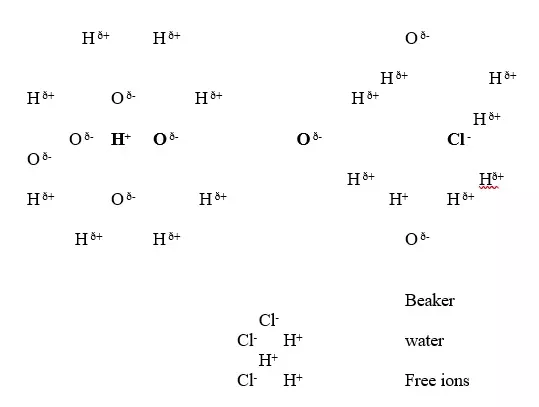Note:Water is polar .It is made up of :
Oxygen atom is partially negative and two hydrogen atoms which are partially positive.
They surround the free H+ and Cl- ions.
A non polar solvent is one which dissolved non-polar substances and covalent compounds.
If a polar ionic compound is dissolved in non-polar solvent ,it does not ionize/dissociate into free ions as below: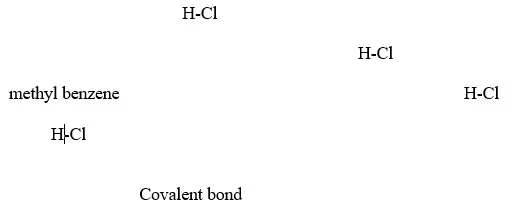7. Some acids and bases are strong while others are weak.
(a) A strong acid/base is one which is fully/wholly/completely dissociated / ionized into many free H+ /OH- ions i.e. I. Strong acids exists more as free H+ ions than molecules. e.g.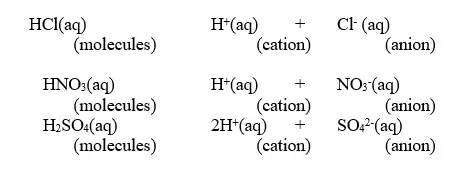II. Strong bases/alkalis exists more as free OH- ions than molecules. e.g.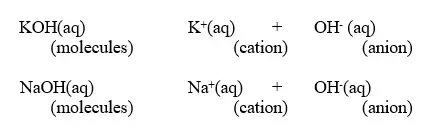(b) A weak base/acid is one which is partially /partly dissociated /ionized in water into free OH- (aq) and H+(aq) ions. I. Weak acids exists more as molecules than as free H+ ions. e.g.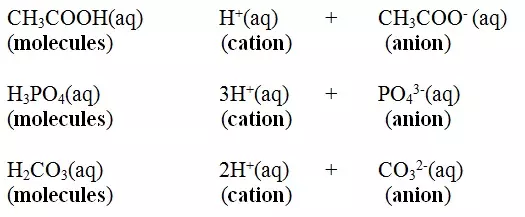II. Weak bases/alkalis exists more as molecules than free OH- ions. e.g.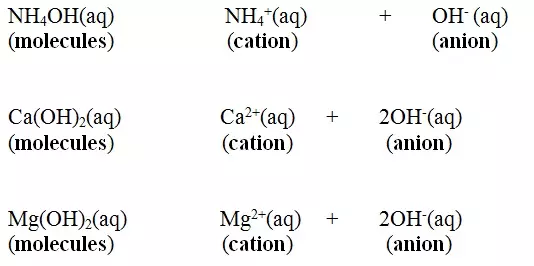8. The concentration of an acid/base/alkali is based on the number of moles of acid/bases dissolved in a decimeter(litre)of the solution.
An acid/base/alkali with more acid/base/alkali in a decimeter(litre) of solution is said to be concentrated while that with less is said to be dilute.
9. (a) (i)strong acids have pH 1/2/3 while weak acids have high pH 4/5/6.
(ii) a neutral solution have pH 7.
(iii) strong alkalis/bases have pH 12/13/14 while weak bases/alkalis have pH 11/10 /9 / 8.
(b) pH is a measure of H+(aq) concentration in a solution.
The higher the H+(aq)ions concentration ;
The higher the acidity
The lower the pH
The lower the concentration of OH-(aq)
The lower the alkalinity
- At pH 7 , a solution has equal concentration of H+(aq) and OH-(aq).
- Beyond pH 7,the concentration of the OH-(aq) increases as the H+(aq) ions decreases.
10. (a) When acids /bases dissolve in water, the ions present in the solution conduct electricity.
The more the dissociation the higher the yield of ions and the greater the electrical conductivity of the solution. A compound that conducts electricity in an electrolyte and thus a compound showing high electrical conductivity is a strong electrolyte while a compound showing low electrical conductivity is a weak electrolyte. (b) Practically, a bright light on a bulb ,a high voltage reading from a voltmeter high ammeter reading from an ammeter,
a big deflection on a galvanometer is an indicator of strong electrolyte(acid/base) and the opposite for weak electrolytes(acids/base).
11. Some compounds exhibit/show both properties of acids and bases/alkalis.
- A substance that reacts with both acids and bases is said to be amphotellic.
- The examples below show the amphotellic properties of:

### (a) Zinc (II)oxide(ZnO) and Zinc hydroxide(Zn(OH)2)

(i)When ½ spatula full of Zinc(II)oxide is placed in a boiling tube containing 10cm3 of either 2M nitric(V)acid or 2M sodium hydroxide hydroxide solution, it dissolves on both the acid and the alkali/base to form a colourless solution. i.e.
(i) When reacting with nitric(V)acid, the oxide shows basic properties by reacting with an acid to form a simple salt and water only.
Basic oxide + Acid → salt + water
Examples:
Chemical equation
ZnO(s) + 2HNO3(aq) → Zn(NO3) 2 (aq) + H2O(l)
ZnO(s) + 2HCl(aq) → ZnCl2 (aq) + H2O(l)
ZnO(s) + H2SO4(aq) → ZnSO4 (aq) + H2O(l)
Ionic equation
ZnO(s) + 2H+ (aq) → Zn2+ (aq) + H2O(l)
(ii) When reacting with sodium hydroxide, the oxide shows acidic properties by reacting with a base to form a complex salt.
Basic oxide + Base/alkali + Water → Complex salt
Examples:
Chemical equation
1. When Zinc oxide is reacted with sodium hydroxide the complex salt is sodium tetrahydroxozincate(II) complex salt.
ZnO(s) + 2NaOH(aq) + H2O(l) → Na2Zn(OH)4(aq)
2. When Zinc oxide is reacted with potassium hydroxide the complex salt is potassium tetrahydroxozincate(II) complex salt.
ZnO(s) + 2KOH(aq) + H2O(l) → K2Zn(OH)4(aq)
Ionic equation
ZnO(s) + 2OH-(aq) + H2O(l) → 2[Zn(OH)4]2- (aq)
(ii) When Zinc(II)hydroxide is placed in a boiling tube containing 10cm3 of either 2M nitric(V)acid or 2M sodium hydroxide hydroxide solution, it dissolves on both the acid and the alkali/base to form a colourless solution. i.e.
(i) When reacting with nitric(V)acid, the hydroxide shows basic properties. It reacts with an acid to form a simple salt and water only.
Basic hydroxide + Acid → salt + water
Examples:
Chemical equation

Zn(OH)2 (s) + 2HNO3(aq) → Zn(NO3) 2 (aq) + 2H2O(l)
Zn(OH)2 (s) + 2HCl(aq) → ZnCl2 (aq) + 2H2O(l)
Zn(OH)2 (s) + H2SO4(aq) → ZnSO4 (aq) + 2H2O(l)
Ionic equation
Zn(OH) 2 (s) + 2H+ (aq) → Zn2+ (aq) + 2H2O(l)
(ii) When reacting with sodium hydroxide, the hydroxide showsacidic properties by reacting with a base to form a complex salt.
Basic hydroxide + Base/alkali → Complex salt
Examples:
Chemical equation

1. When Zinc hydroxide is reacted with sodium hydroxide the complex salt is sodium tetrahydroxozincate(II) complex salt.
Zn(OH)2 (s) + 2NaOH(aq) → Na2Zn(OH)4(aq)
2. When Zinc hydroxide is reacted with potassium hydroxide the complex salt is potassium tetrahydroxozincate(II) complex salt.
Zn(OH)2 (s) + 2KOH(aq) → K2Zn(OH)4(aq)
Ionic equation
Zn(OH)2 (s) + 2OH-(aq) → 2[Zn(OH)4]2- (aq)

(i) When ½ spatula full of Lead(II)oxide is placed in a boiling tube containing 10cm3 of either 2M nitric(V)acid or 2M sodium hydroxide hydroxide solution, it dissolves on both the acid and the alkali/base to form a colourless solution. i.e.
(i) When reacting with nitric(V)acid, the oxide shows basic properties by reacting with an acid to form a simple salt and water only. All other Lead salts are insoluble.
Chemical equation
PbO(s) + 2HNO3(aq) → Pb(NO3) 2 (aq) + H2O(l)
Ionic equation
PbO(s) + 2H+ (aq) → Pb2+ (aq) + H2O(l)
(ii) When reacting with sodium hydroxide, the oxide shows acidic properties by reacting with a base to form a complex salt.
Chemical equation
1. When Lead(II) oxide is reacted with sodium hydroxide the complex salt is sodium tetrahydroxoplumbate(II) complex salt.
PbO(s) + 2NaOH(aq) + H2O(l) → Na2Pb(OH)4(aq)
2. When Lead(II) oxide is reacted with potassium hydroxide the complex salt is potassium tetrahydroxoplumbate(II) complex salt.
PbO(s) + 2KOH(aq) + H2O(l) → K2Pb(OH)4(aq)
Ionic equation
PbO(s) + 2OH-(aq) + H2O(l) → 2[Pb(OH)4]2- (aq)
(ii) When Lead(II)hydroxide is placed in a boiling tube containing 10cm3 of either 2M nitric(V)acid or 2M sodium hydroxide hydroxide solution, it dissolves on both the acid and the alkali/base to form a colourless solution. i.e.
(i) when reacting with nitric(V)acid, the hydroxide shows basic properties. It reacts with the acid to form a simple salt and water only.
Chemical equation
Pb(OH)2 (s) + 2HNO3(aq) → Pb(NO3) 2 (aq) + 2H2O(l)
Ionic equation
Pb(OH)2 (s) + 2H+ (aq) → Pb2+(aq) + 2H2O(l)
(ii) when reacting with sodium hydroxide, the hydroxide shows acidic properties. It reacts with a base to form a complex salt.
Chemical equation
1. When Lead(II) hydroxide is reacted with sodium hydroxide the complex salt is sodium tetrahydroxoplumbate(II) complex salt.
Pb(OH)2 (s) + 2NaOH(aq) → Na2Pb(OH)4(aq)
2. When Lead(II) hydroxide is reacted with potassium hydroxide the complex salt is potassium tetrahydroxoplumbate(II) complex salt.
Pb(OH)2 (s) + 2KOH(aq) → K2Pb(OH)4(aq)
Ionic equation
Pb(OH)2 (s) + 2OH-(aq) → 2[Pb(OH)4]2- (aq)

### (c) Aluminium(III)oxide(Al2O3) and Aluminium(III)hydroxide(Al(OH)3)

(i)When ½ spatula full of Aluminium(III)oxide is placed in a boiling tube containing 10cm3 of either 2M nitric(V)acid or 2M sodium hydroxide hydroxide solution, it dissolves on both the acid and the alkali/base to form a colourless solution. i.e.
(i) when reacting with nitric(V)acid, the oxide shows basic properties by reacting with an acid to form a simple salt and water only.
Chemical equation
Al2O3 (s) + 6HNO3(aq) → Al(NO3)3 (aq) + 3H2O(l)
Al2O3 (s) + 6HCl(aq) → AlCl3 (aq) + 3H2O(l)
Al2O3 (s) + 3H2SO4(aq) → Al2(SO4)3 (aq) + 3H2O(l)
Ionic equation
Al2O3 (s) + 3H+ (aq) → Al3+ (aq) + 3H2O(l)
(ii) When reacting with sodium hydroxide, the oxide shows acidic properties by reacting with a base to form a complex salt.
Chemical equation
1. When Aluminium(III) oxide is reacted with sodium hydroxide the complex salt is sodium tetrahydroxoaluminate(III) complex salt.
Al2O3 (s) + 2NaOH(aq) + 3H2O(l) → 2NaAl(OH)4(aq)
2. When Aluminium(III) oxide is reacted with potassium hydroxide the complex salt is potassium tetrahydroxoaluminate(II) complex salt.
Al2O3 (s) + 2KOH(aq) + 3H2O(l) → 2NaAl(OH)4(aq)
Ionic equation
Al2O3 (s) + 2OH-(aq) + 3H2O(l) → 2[Al(OH)4]- (aq)
(ii) When Aluminium(III)hydroxide is placed in a boiling tube containing 10cm3 of either 2M nitric(V)acid or 2M sodium hydroxide hydroxide solution, it dissolves on both the acid and the alkali/base to form a colourless solution. i.e.
(i) when reacting with nitric(V)acid, the hydroxide shows basic properties. It reacts with the acid to form a simple salt and water only.
Chemical equation
Al(OH)3 (s) + 3HNO3(aq) → Al(NO3)3 (aq) + 3H2O(l)
Al(OH)3 (s) + 3HCl(aq) → AlCl3 (aq) + 3H2O(l)
2Al(OH)3 (s) + 3H2SO4(aq) → Al2(SO4)3 (aq) + 3H2O(l)
Ionic equation
Al(OH)3 (s) + 3H+ (aq) → Al3+ (aq) + 3H2O(l)
(ii) when reacting with sodium hydroxide, the hydroxide shows acidic properties. It reacts with a base to form a complex salt.
Chemical equation
1. When aluminium(III) hydroxide is reacted with sodium hydroxide the complex salt is sodium tetrahydroxoaluminate(III) complex salt.
Al(OH)3 (s) + NaOH(aq) → NaAl(OH)4(aq)
2.When aluminium(III) hydroxide is reacted with potassium hydroxide the complex salt is potassium tetrahydroxoaluminate(III) complex salt.
Al(OH)3 (s) + KOH(aq) → KAl(OH)4(aq)
Ionic equation
Al(OH)3 (s) + OH-(aq) → [Al(OH)4]- (aq)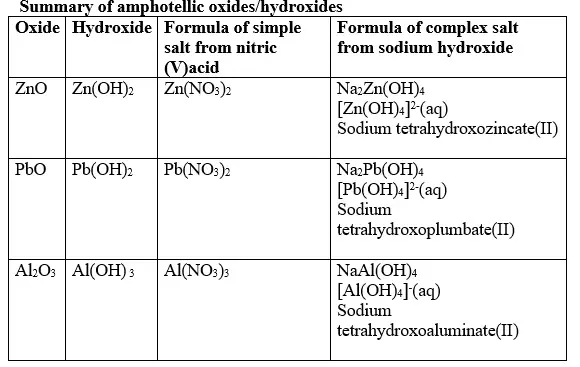12. (a) A salt is an ionic compound formed when the cation from a base combine with the anion derived from an acid.
A salt is therefore formed when the hydrogen ions in an acid are replaced wholly/fully or partially/partly ,directly or indirectly by a metal or ammonium radical.
(b) The number of ionizable/replaceable hydrogen in an acid is called basicity of an acid.
Some acids are therefore:
(i)Monobasic acids generally denoted HX e.g. HCl, HNO3,HCOOH,CH3COOH.
(ii)Dibasic acids ; generally denoted H2X e.g. H2SO4, H2SO3, H2CO3,HOOCOOH.
(iii)Tribasic acids ; generally denoted H4X e.g. H3PO4.
(c) Some salts are normal salts while other are acid salts.
(i)A normal salt is formed when all the ionizable /replaceable hydrogen in an acid is replaced by a metal or metallic /ammonium radical.
(ii)An acid salt is formed when part/portion the ionizable /replaceable hydrogen in an acid is replaced by a metal or metallic /ammonium radical.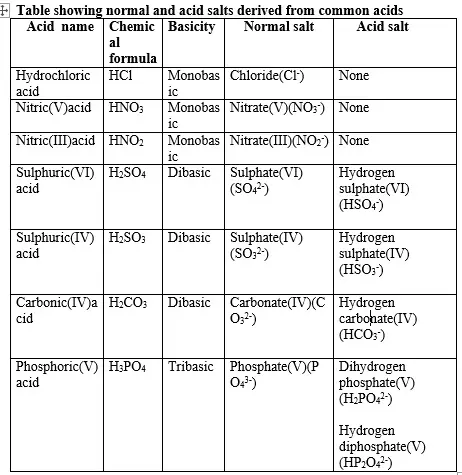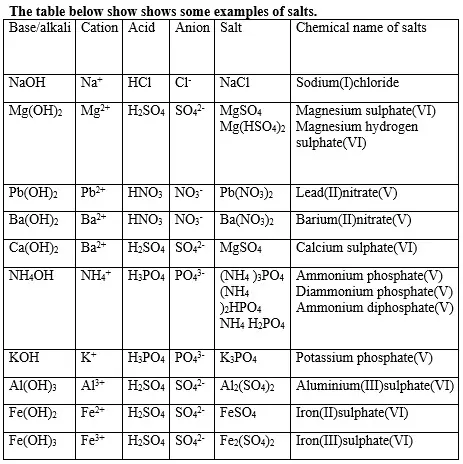(d) Some salts undergo hygroscopy, deliquescence and efflorescence.
(i) Hygroscopic salts /compounds are those that absorb water from the atmosphere but do not form a solution.
Some salts which are hygroscopic include anhydrous copper(II)sulphate(VI), anhydrous cobalt(II)chloride, potassium nitrate(V) common table salt.
(ii)Deliquescent salts /compounds are those that absorb water from the atmosphere and form a solution.
Some salts which are deliquescent include: Sodium nitrate(V),Calcium chloride, Sodium hydroxide, Iron(II)chloride, Magnesium chloride.
(iii)Efflorescent salts/compounds are those that lose their water of crystallization to the atmosphere.
Some salts which effloresces include: sodium carbonate decahydrate, Iron(II)sulphate(VI)heptahydrate, sodium sulphate (VI)decahydrate.
(e)Some salts contain water of crystallization.They are hydrated.Others do not contain water of crystallization. They are anhydrous.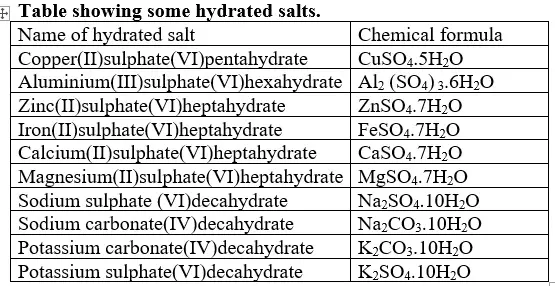(f)Some salts exist as a simple salt while some as complex salts. Below are some complex salts.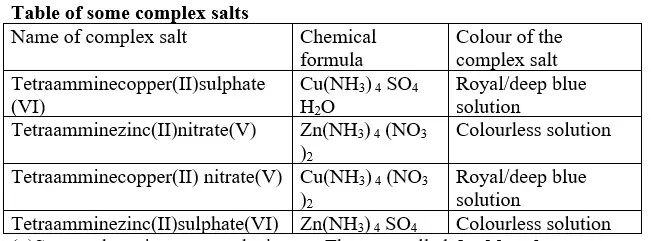(g)Some salts exist as two salts in one. They are called double salts.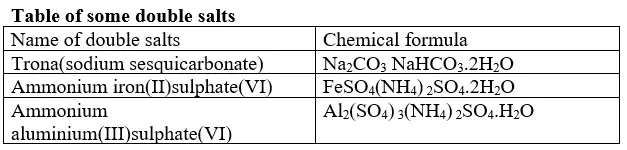(h)Some salts dissolve in water to form a solution. They are said to be soluble. Others do not dissolve in water. They form a suspension/precipitate in water.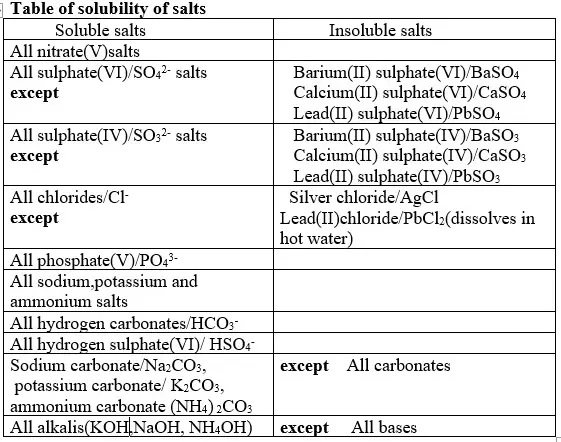13. Salts can be prepared in a school laboratory by a method that uses its solubility in water.
(a) Soluble salts may be prepared by using any of the following methods:

#### (i) Direct displacement/reaction of a metal with an acid.

- Excess of the metal must be used to ensure all the acid has reacted.
- When effervescence/bubbling /fizzing has stopped ,excess metal is filtered.
- The filtrate is heated to concentrate then allowed to crystallize.
- Washing with distilled water then drying between filter papers produces a sample crystal of the salt. i.e.
M(s) + H2X → MX(aq) + H2(g)
Examples
Mg(s) + H2SO4(aq) → MgSO4 (aq) + H2(g)
Zn(s) + H2SO4(aq) → ZnSO4 (aq) + H2(g)
Pb(s) + 2HNO3(aq) → Pb(NO3) 2(aq) + H2(g)
Ca(s) + 2HNO3(aq) → Ca(NO3) 2(aq) + H2(g)
Mg(s) + 2HNO3(aq) → Mg(NO3) 2(aq) + H2(g)
Mg(s) + 2HCl(aq) → MgCl2(aq) + H2(g)
Zn(s) + 2HCl(aq) → ZnCl2(aq) + H2(g)

#### (ii) Reaction of an insoluble base with an acid

- By adding an insoluble base (oxide/hydroxide )to a dilute acid until no more dissolves, in the acid,a salt and water are formed.
- Excess of the base is filtered off.
- The filtrate is heated to concentrate ,allowed to crystallize then washed with distilled water before drying between filter papers e.g.
PbO(s) + 2HNO3(aq) → Pb(NO3) 2(aq) + H2O (l)
Pb(OH)2(s) + 2HNO3(aq) → Pb(NO3) 2(aq) + 2H2O (l)
CaO (s) + 2HNO3(aq) → Ca(NO3) 2(aq) + H2O (l)
MgO (s) + 2HNO3(aq) → Mg(NO3) 2(aq) + H2O (l)
MgO (s) + 2HCl(aq) → MgCl2(aq) + H2O (l)
ZnO (s) + 2HCl(aq) → ZnCl2(aq) + H2O (l)
Zn(OH)2(s) + 2HNO3(aq) → Zn(NO3) 2(aq) + 2H2O (l)
CuO (s) + 2HCl(aq) → CuCl 2(aq) + H2O (l)
CuO (s) + H2SO4(aq) → CuSO4(aq) + H2O (l)
Ag2O(s) + 2HNO3(aq) → 2AgNO3(aq) + H2O (l)
Na2O(s) + 2HNO3(aq) → 2NaNO3(aq) + H2O (l)

#### (iii) Reaction of insoluble /soluble carbonate /hydrogen carbonate with an acid.

- By adding an excess of a soluble /insoluble carbonate or hydrogen carbonate to a dilute acid, effervescence /fizzing/bubbling out of carbon(IV)oxide gas shows the reaction is taking place.
- When effervescence /fizzing/bubbling out of the gas is over, excess of the insoluble carbonate is filtered off.
- The filtrate is heated to concentrate ,allowed to crystallize then washed with distilled water before drying between filter paper papers e.g.
PbCO3 (s) + 2HNO3(aq) → Pb(NO3) 2(aq) + H2O (l)+ CO2(g)
ZnCO3 (s) + 2HNO3(aq) → Zn(NO3) 2(aq) + H2O (l)+ CO2(g)
CaCO3 (s) + 2HNO3(aq) → Ca(NO3) 2(aq) + H2O (l)+ CO2(g)
MgCO3 (s) + H2SO4(aq) → MgSO4(aq) + H2O (l)+ CO2(g)
CuCO3 (s) + H2SO4(aq) → CuSO4(aq) + H2O (l) + CO2(g)
Ag2CO3 (s) + 2HNO3(aq) → 2AgNO3(aq) + H2O (l) + CO2(g)
Na2CO3 (s) + 2HNO3(aq) → 2NaNO3(aq) + H2O (l) + CO2(g)
K2CO3 (s) + 2HCl(aq) → 2KCl(aq) + H2O (l) + CO2(g)
NaHCO3 (s) + HNO3(aq) → NaNO3(aq) + H2O (l) + CO2(g)
KHCO3 (s) + HCl(aq) → KCl(aq) + H2O (l) + CO2(g)

#### (iv) Neutralization/reaction of soluble base/alkali with dilute acid

By adding an acid to a burette into a known volume of an alkali with 2-3 drops of an indicator, the colour of the indicator changes when the acid has completely reacted with an alkali at the end point.
The procedure is then repeated without the indicator .The solution mixture is then heated to concentrate , allowed to crystallize ,washed with distilled water before drying with filter papers. e.g.
NaOH (aq) + HNO3(aq) → NaNO3(aq) + H2O (l)
KOH (aq) + HNO3(aq) → KNO3(aq) + H2O (l)
KOH (aq) + HCl(aq) → KCl(aq) + H2O (l)
2KOH (aq) + H2SO4(aq) → K2SO4(aq) + 2H2O (l) 2NH4OH (aq) + H2SO4(aq) → (NH4)2SO4(aq) + 2H2O (l)
NH4OH (aq) + HNO3(aq) → NH4NO3(aq) + H2O (l)

#### (iv) Direct synthesis/combination.

When a metal burn in a gas jar containing a non metal, the two directly combine to form a salt. e.g.
2Na(s) + Cl2(g) → 2NaCl(s)
2K(s) + Cl2(g) → 2KCl(s)
Mg(s) + Cl2(g) → MgCl2 (s)
Ca(s) + Cl2(g) → CaCl2 (s)
- Some salts once formed undergo sublimation and hydrolysis.
- Care should be taken to avoid water/moisture into the reaction flask during their preparation.
- Such salts include aluminium(III)chloride(AlCl3) and iron (III)chloride(FeCl3).
1. Heated aluminium foil reacts with chlorine to form aluminium(III)chloride that sublimes away from the source of heating then deposited as solid again.
2Al(s) + 3Cl2(g) → 2AlCl3 (s/g)
Once formed aluminium(III)chloride hydrolyses/reacts with water vapour / moisture present to form aluminium hydroxide solution and highly acidic fumes of hydrogen chloride gas.
AlCl3(s)+ 3H2O(g) → Al(OH)3 (aq) + 3HCl(g)
2. Heated iron filings reacts with chlorine to form iron(III)chloride that sublimes away from the source of heating then deposited as solid again.
2Fe(s) + 3Cl2(g) → 2FeCl3 (s/g)
Once formed , aluminium(III)chloride hydrolyses/reacts with water vapour / moisture present to form aluminium hydroxide solution and highly acidic fumes of hydrogen chloride gas.
FeCl3(s)+ 3H2O(g) → Fe(OH)3 (aq) + 3HCl(g)
(b) Insoluble salts can be prepared by reacting two suitable soluble salts to form one soluble and one insoluble.
- This is called double decomposition or precipitation.
- The mixture is filtered and the residue is washed with distilled water then dried.

CuSO4(aq) + Na2CO3 (aq) → CuCO3 (s) + Na2SO4(aq)
BaCl2(aq) + K2SO4 (aq) → BaSO4 (s) + 2KCl (aq)
Pb(NO3)2(aq) + K2SO4 (aq) → PbSO4 (s) + 2KNO3 (aq)
2AgNO3(aq) + MgCl2 (aq) → 2AgCl(s) + Mg(NO3)2 (aq)
Pb(NO3)2(aq) + (NH4)2SO4 (aq) → PbSO4 (s) + 2NH4NO3(aq)
BaCl2(aq) + K2SO3 (aq) → BaSO4 (s) + 2KCl (aq)
14. Salts may lose their water of crystallization , decompose ,melt or sublime on heating on a Bunsen burner flame.
- The following shows the behavior of some salts on heating gently /or strongly in a laboratory school burner:

#### (a) Effect of heat on Chlorides.

All chlorides have very high melting and boiling points and therefore are not affected by laboratory heating except ammonium chloride. Ammonium chloride sublimes on gentle heating. It dissociate into the constituent ammonia and hydrogen chloride gases on strong heating.
NH4Cl(s) [(sublimation)] NH4Cl(g) : NH3(g) + HCl(g) [(dissociation)]

#### (b) Effect of heat on nitrate .

(i) Potassium nitrate(V)/KNO3 and sodium nitrate(V)/NaNO3 decompose on heating to form Potassium nitrate(III)/KNO2 and sodium nitrate(III)/NaNO2 and producing Oxygen gas in each case.
2KNO3 (s) → 2KNO2(s) + O2(g)
2NaNO3 (s) → 2NaNO2(s) + O2(g)
2Mg(NO3)2(s) → 2MgO(s) + 4NO2(g) + O2(g)
2Zn(NO3)2(s) → 2ZnO(s) + 4NO2(g) + O2(g)
2Pb(NO3)2(s) → 2PbO(s) + 4NO2(g) + O2(g)
2Cu(NO3)2(s) → 2CuO(s) + 4NO2(g) + O2(g)
2Fe(NO3)2(s) → 2FeO(s) + 4NO2(g) + O2(g)
(iii) Silver(I)nitrate(V) and mercury(II) nitrate(V) are lowest in the reactivity series. They decompose on heating to form the metal(silver and mercury)and the Nitrogen(IV)oxide and oxygen gas. i.e.
2AgNO3(s) → 2Ag (s) + 2NO2(g) + O2(g)
2Hg(NO3)2 (s) → 2Hg (s) + 4NO2(g) + O2(g)
(iv) Ammonium nitrate(V) and Ammonium nitrate(III) decompose on heating to Nitrogen(I)oxide(relights/rekindles glowing splint) and nitrogen gas respectively.Water is also formed.i.e.
NH4NO3(s) → N2O (g) + H2O(l)
NH4NO2(s) → N2(g) + H2O(l)

#### (c) Effect of heat on Sulphate

Only Iron(II)sulphate(VI), Iron(III)sulphate(VI) and copper(II)sulphate(VI) decompose on heating. They form the oxide, and produce highly acidic fumes of acidic sulphur(IV)oxide gas.
2FeSO4 (s) → Fe2O3(s) + SO3(g) + SO2(g)
Fe2(SO4) 3(s) → Fe2O3(s) + SO3(g)
CuSO4 (s) → CuO(s) + SO3(g)

#### (d) Effect of heat on carbonates(IV) and hydrogen carbonate(IV).

(i)Sodium carbonate(IV)and potassium carbonate(IV)do not decompose on heating.
(ii)Heavy metal nitrate(IV)salts decompose on heating to form the oxide and produce carbon(IV)oxide gas. Carbon (IV)oxide gas forms a white precipitate when bubbled in lime water. The white precipitate dissolves if the gas is in excess. e.g.
CuCO3 (s) → CuO(s) + CO2(g)
CaCO3 (s) → CaO(s) + CO2(g)
PbCO3 (s) → PbO(s) + CO2(g)
FeCO3 (s) → FeO(s) + CO2(g)
ZnCO3 (s) → ZnO(s) + CO2(g)
(iii)Sodium hydrogen carbonate(IV) and Potassium hydrogen carbonate(IV)decompose on heating to give the corresponding carbonate (IV) and form water and carbon(IV)oxide gas. i.e.
2NaHCO3(s) → Na2CO3(s) + CO2(g) + H2O(l)
2KHCO3(s) → K2CO3(s) + CO2(g) + H2O(l)
(iv) Calcium hydrogen carbonate (IV) and Magnesium hydrogen carbonate(IV) decompose on heating to give the corresponding carbonate (IV) and form water and carbon(IV)oxide gas. i. e.
Ca(HCO3)2(aq) → CaCO3(s) + CO2(g) + H2O(l)
Mg(HCO3)2(aq) → MgCO3(s) + CO2(g) + H2O(l)
15. Salts contain cation(positively charged ion) and anions(negatively charged ion).When dissolved in polar solvents/water.
- The cation and anion in a salt is determined/known usually by precipitation of the salt using a precipitating reagent.
- The colour of the precipitate is a basis of qualitative analysis of a compound.
16. Qualitative analysis is the process of identifying an unknown compound /salt by identifying the unique qualities of the salt/compound.
It involves some of the following processes.

## (a) Reaction of cation with sodium/potassium hydroxide solution.

- Both sodium/potassium hydroxide solutions are precipitating reagents.
- The alkalis produce unique colour of a precipitate/suspension when a few/three drops is added and then excess alkali is added to unknown salt/compound solution.
NB: Potassium hydroxide is not commonly used because it is more expensive than sodium hydroxide.
- The table below shows the observations, inferences / deductions and explanations from the following test tube experiments:

#### Procedure

Put about 2cm3 of MgCl2, CaCl2, AlCl3, NaCl, KCl, FeSO4, Fe2(SO4)3, CuSO4, ZnSO4NH4NO3, Pb(NO3)2, Ba(NO3)2 each into separate test tubes. Add three drops of 2M sodium hydroxide solution then excess (2/3 the length of a standard test tube).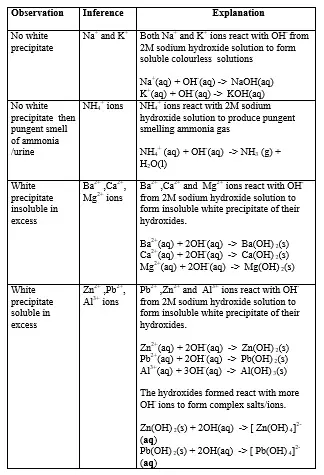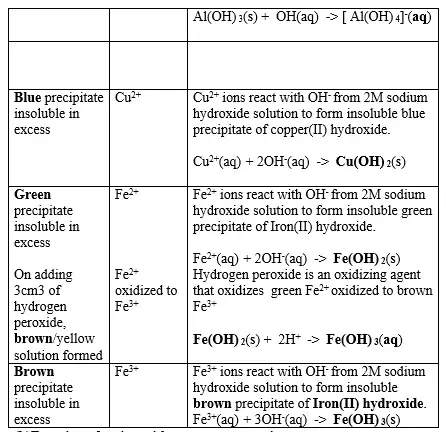## b. Reaction of cation with aqueous ammonia.

- Aqueous ammonia precipitating reagent that can be used to identify the cations present in a salt.
- Like NaOH/KOH the OH- ion in NH4OH react with the cation to form a characteristic hydroxide .
- Below are the observations ,inferences and explanations of the reactions of aqueous ammonia with salts from the following test tube reactions.
Experiment
- Put about 2cm3 of MgCl2, CaCl2, AlCl3, NaCl, KCl, FeSO4, Fe2(SO4)3, CuSO4, ZnSO4, NH4NO3, Pb(NO3)2, Ba(NO3)2 each into separate test tubes. - Add three drops of 2M aqueous ammonia then excess (2/3 the length of a standard test tube).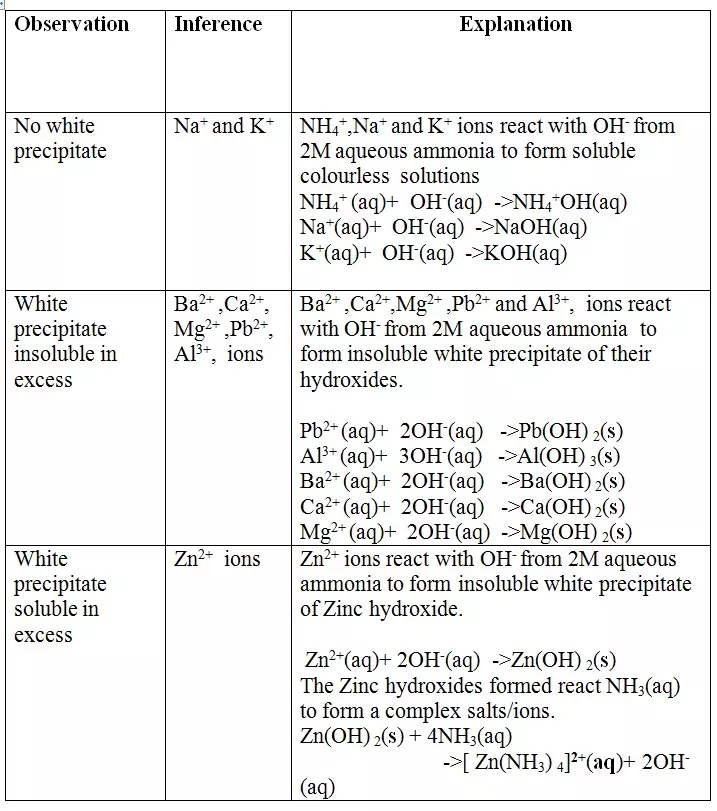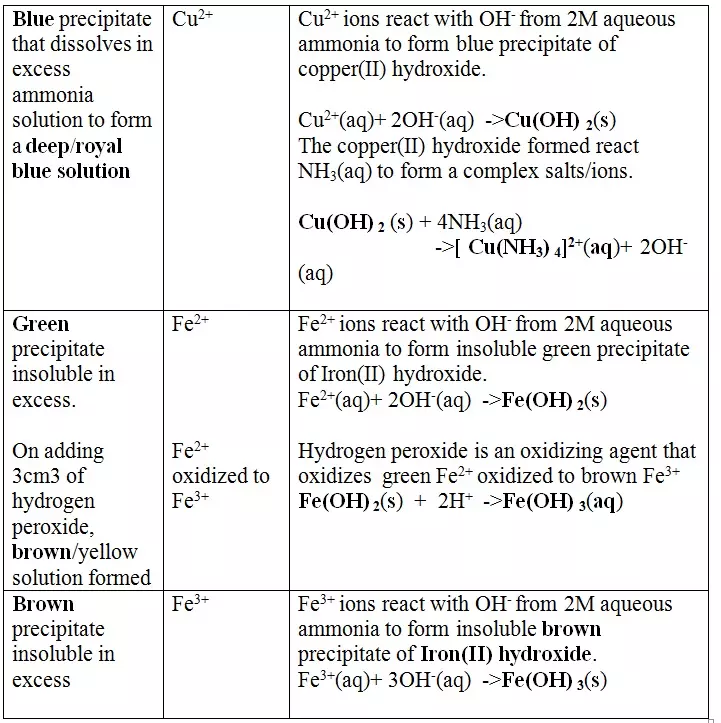Note
(i) Only Zn2+ ions/salts form a white precipitate that dissolve in excess of both 2M sodium hydroxide and 2M aqueous ammonia.
(ii) Pb2+ and Al3+ ions/salts form a white precipitate that dissolve in excess of 2M sodium hydroxide but not in 2M aqueous ammonia.
(iii) Cu2+ ions/salts form a blue precipitate that dissolve to form a deep/royal blue solution in excess of 2M aqueous ammonia but only blue insoluble precipitate in 2M sodium hydroxide

## c. Reaction of cation with Chloride (Cl-) ions.

All chlorides are soluble in water except Silver chloride and Lead (II)chloride (That dissolve in hot water).When a soluble chloride like NaCl, KCl, NH4Cl is added to about 2cm3 of a salt containing Ag+ or Pb2+ions a white precipitate of AgCl or PbCl2 is formed. The following test tube reactions illustrate the above. Experiment
Put about 2cm3 of silver nitrate(V) and Lead(II) nitrate(V) solution into separate test tubes. Add five drops of NaCl /KCl / NH4Cl/HCl. Heat to boil.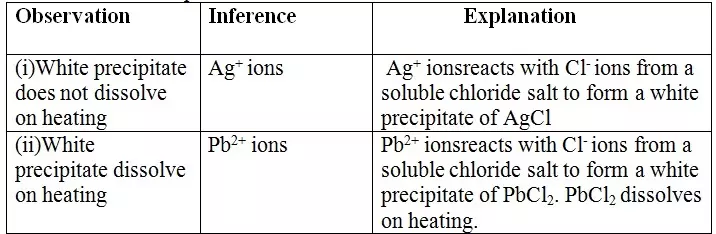Note
- Both Pb2+ and Al3+ ions forms an insoluble white precipitate in excess aqueous ammonia.
- A white precipitate on adding Cl- ions/salts shows Pb2+.
- No white precipitate on adding Cl- ions/salts shows Al3+.
- Adding a chloride/ Cl- ions/salts can thus be used to separate the identity of Al3+ and Pb2+.

## (d) Reaction of cation with sulphate(VI)/SO42- and sulphate(IV)/SO32- ions

All sulphate(VI) and sulphate(IV)/SO32- ions/salts are soluble/dissolve in water except Calcium sulphate(VI)/CaSO4, Calcium sulphate(IV)/CaSO3, Barium sulphate(VI)/BaSO4, Barium sulphate(IV)/BaSO3, Lead(II) sulphate(VI)/PbSO4 and Lead(II) sulphate(IV)/PbSO3.
When a soluble sulphate(VI)/SO42- salt like Na2SO4, H2SO4, (NH4)2SO4 or Na2SO3 is added to a salt containing Ca2+, Pb2+, Ba2+ ions, a white precipitate is formed.
The following test tube experiments illustrate the above.

#### Procedure

Place about 2cm3 of Ca(NO3)2, Ba(NO3)2, BaCl2 and Pb(NO3)2, in separate boiling tubes. Add six drops of sulphuric(VI)acid /sodium sulphate(VI)/ammonium sulphate(VI)solution. Repeat with six drops of sodium sulphate(IV).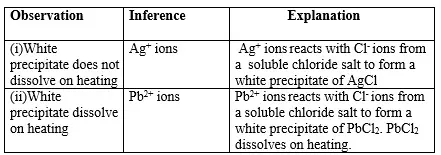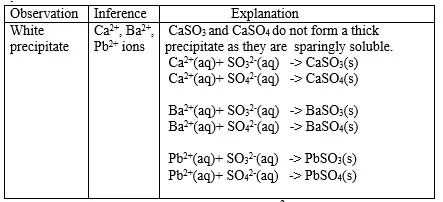## (e) Reaction of cation with carbonate(IV)/CO32- ions

All carbonate salts are insoluble except sodium/potassium carbonate(IV) and ammonium carbonate(IV).
They dissociate /ionize to release CO32- ions. CO32- ions produce a white precipitate when the soluble carbonate salts is added to any metallic cation.

#### Procedure

- Place about 2cm3 of Ca(NO3)2, Ba(NO3)2, MgCl2 ,Pb(NO3)2 andZnSO4 in separate boiling tubes.
- Add six drops of Potassium /sodium carbonate(IV)/ ammonium carbonate (IV)solution.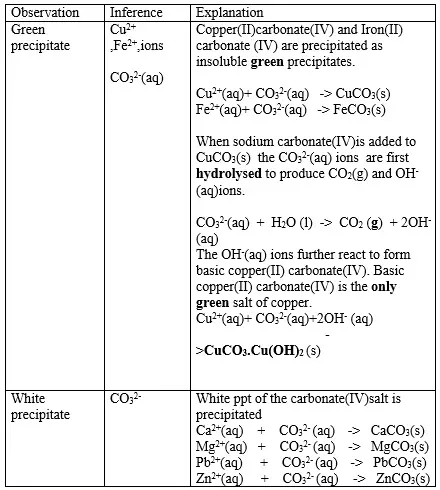Note
(i)Iron(III)carbonate(IV) does not exist.
(ii)Copper(II)Carbonate(IV) exist only as the basic CuCO3.Cu(OH) 2
(iii)Both BaCO3 and BaSO3 are insoluble white precipitate. If hydrochloric acid is added to the white precipitate;
• BaCO3 produces CO2 gas. When bubbled/directed into lime water solution,a white precipitate is formed.
• BaSO3 produces SO2 gas. When bubbled/directed into orange acidified potassium dichromate(VI) solution, it turns to green/decolorizes acidified potassium manganate(VII).

## (f) Reaction of cation with sulphide / S2- ions

All sulphides are insoluble black solids/precipitates except sodium sulphide/ Na2S/ potassium sulphide/K2S.When a few/3drops of the soluble sulphide is added to a metal cation/salt, a black precipitate is formed.

#### Procedure

Place about 2cm3 of Cu(NO3)2, FeSO4, MgCl2,Pb(NO3)2 and ZnSO4 in separate boiling tubes.
Add six drops of Potassium /sodium sulphide solution.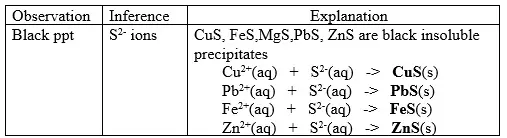The maximum mass of salt/solid/solute that dissolve in 100g of solvent/water at a specified temperature is called solubility of a salt.
When no more solute can dissolve in a given amount of solvent at a specified temperature, a saturated solution is formed.
For some salts, on heating, more of the salt/solid/solute dissolve in the saturated solution to form a super saturated solution.
The solubility of a salt is thus calculated from the formula.
Solubility = Mass of solute/salt/solid x 100
Mass/volume of water/solvent
20. Solubility and solubility curves are therefore used
(i) to know the effect of temperature on the solubility of a salt.
(ii)to fractional crystallize two soluble salts by applying their differences in solubility at different temperatures.
(iii)determine the mass of crystal that is obtained from crystallization.
21.Natural fractional crystallization takes place in Kenya/East Africa at:
(i) Lake Magadi during extraction of soda ash(Sodium carbonate) from Trona(sodium sesquicarbonate).
(ii) Ngomeni near Malindi at the Indian Ocean Coastline during the extraction of common salt(sodium chloride).
22.Extraction of soda ash from Lake Magadi in Kenya
Rain water drains underground in the great rift valley and percolate underground where it is heated geothermically.
The hot water dissolves underground soluble sodium compounds and comes out on the surface as alkaline springs which are found around the edges of Lake Magadi in Kenya.
Temperatures around the lake are very high (30-40oC) during the day.
The solubility of trona decrease with increase in temperature therefore solid crystals of trona grows on top of the lake (upto or more than 30metres thick).
A bucket dredger mines the trona which is then crushed ,mixed with lake liquor and pumped to washery plant where it is further refined to a green granular product called CRS.
The CRS is then heated to chemically decompose trona to soda ash(Sodium carbonate).
Chemical equation
2Na2CO3.NaHCO3.2H2O(s) → 3Na2CO3 (s) + CO2(g) + 5H2O(l)
Soda ash(Sodium carbonate) is then stored .It is called Magadi Soda. Magadi Soda is used :
(i) make glass
(ii) for making soapless detergents
(iii)softening hard water.
(iv) Common salt is colledcted at night because its solubility decreases with decrease in temperature. It is used as salt lick/feed for animals.
Summary flow diagram showing the extraction of Soda ash from Trona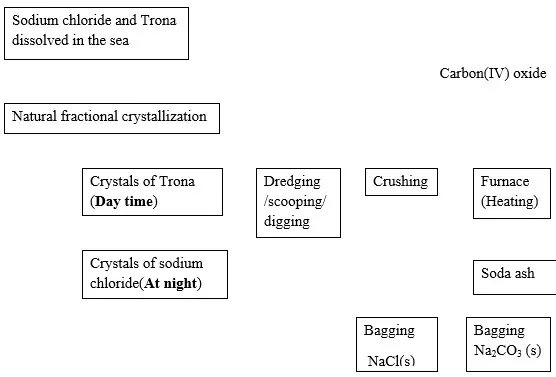23. Extraction of common salt from Indian Ocean at Ngomeni in Kenya.
Oceans are salty.They contain a variety of dissolved salts (about 77% being sodium chloride).
During high tide ,water is collected into shallow pods and allowed to crystallize as evaporation takes place.The pods are constructed in series to increase the rate of evaporation.
At the final pod ,the crystals are scapped together,piled in a heap and washed with brine (concentrated sodium chloride).
It contains MgCl2 and CaCl2. MgCl2 and CaCl2are hygroscopic. They absorb water from the atmosphere and form a solution.
This makes table salt damp/wet on exposure to the atmosphere.
24. Some water form lather easily with soap while others do not.
Water which form lather easily with soap is said to be soft.
Water which do not form lather easily with soap is said to be hard.
Hardness of water is caused by the presence of Ca2+ and Mg2+ ions.
Ca2+ and Mg2+ ions react with soap to form an insoluble grey /white suspension/precipitate called Scum/ curd. Ca2+ and Mg2+ ions in water come from the water sources passing through rocks containing soluble salts of Ca2+ and Mg2+ e.g. Limestone or gypsum.
There are two types of water hardness:
(a)temporary hardness of water
(b)permanent hardness of water

### (a) Temporary hardness of water

Temporary hardness of water is caused by the presence of dissolved calcium hydrogen carbonate/Ca(HCO3)2 and magnesium hydrogen carbonate/Mg(HCO3)2 .
When rain water dissolve carbon(IV) oxide from the air it forms weak carbonic(IV) acid i.e.
CO2(g) + H2O(l) → H2CO3(aq)
When carbonic(IV) acid passes through limestone/dolomite rocks it reacts to form soluble salts i.e.
In limestone areas; H2CO3(aq) + CaCO3(s) → Ca(HCO3)2 (aq)
In dolomite areas; H2CO3(aq) + MgCO3(s) → Mg(HCO3)2 (aq)

### (b) Permanent hardness of water

Permanent hardness of water is caused by the presence of dissolved calcium sulphate(VI)/CaSO4 and magnesium sulphate(VI)/MgSO4 Permanent hardness of water is caused by water dissolving CaSO4 and MgSO4 from ground rocks.
Hardness of water can be removed by the following methods:

### (a)Removing temporary hardness of water

#### (i) Boiling/heating.

Boiling decomposes insoluble calcium hydrogen carbonate/Ca(HCO3)2 and magnesium hydrogen carbonate/Mg(HCO3)2 to insoluble CaCO3 and MgCO3 that precipitate away. i.e
Chemical equation
Ca(HCO3)2(aq) → CaCO3 (s) + CO2(g) + H2O(l)
Mg(HCO3)2(aq) → MgCO3 (s) + CO2(g) + H2O(l)

#### (ii) Adding sodium carbonate (IV) /Washing soda.

Since boiling is expensive on a large scale ,a calculated amount of sodium carbonate decahydrate /Na2CO3.10H2O precipitates insoluble Ca2+(aq) and Mg2+(aq) ions as carbonates to remove both temporary and permanent hardness of water . This a double decomposition reaction where two soluble salts form an insoluble and soluble salt. i.e.
(i) With temporary hard water
Chemical equation
Na2CO3 (aq) + Ca(HCO3)2 (aq) → NaHCO3(aq) + CaCO3 (s)
Na2CO3 (aq) + Mg(HCO3)2 (aq) → NaHCO3(aq) + MgCO3 (s)
Ionic equation
CO32- (aq) + Ca2+ (aq) → CaCO3 (s)
CO32- (aq) + Mg2+ (aq) → MgCO3 (s)
(ii) With permanent hard water
Chemical equation
Na2CO3 (aq) + MgSO4 (aq) → Na2SO4 (aq) + MgCO3 (s)
Na2CO3 (aq) + CaSO4 (aq) → Na2SO4 (aq) + MgCO3 (s)
Ionic equation
CO32- (aq) + Ca2+ (aq) → CaCO3 (s)
CO32- (aq) + Mg2+ (aq) → MgCO3 (s)

#### (iii) Adding calcium (II)hydroxide/Lime water

- Lime water/calcium hydroxide removes only temporary hardness of water from by precipitating insoluble calcium carbonate(IV).
Chemical equation
Ca(OH)2 (aq) + Ca(HCO3)2 (aq) → 2H2O(l) + 2CaCO3 (s)
- Excess of Lime water/calcium hydroxide should not be used because it dissolves again to form soluble calcium hydrogen carbonate(IV) causing the hardness again.

- Aqueous ammonia removes temporary hardness of water by precipitating insoluble calcium carbonate(IV) and magnesium carbonate(IV)
Chemical equation
2NH3(aq) + Ca(HCO32 (aq) → (NH4)2CO3(aq) + CaCO3 (s)
2NH3(aq) + Mg(HCO3)2(aq) → (NH4)2CO3(aq) + MgCO3 (s)

#### (v) Use of ion-exchange permutit

- This method involves packing a chamber with a resin made of insoluble complex of sodium salt called sodium permutit.
- The sodium permutit releases sodium ions that are exchanged with Mg2+ and Ca2+ ions in hard water making the water to be soft. i.e.
Na2X(aq) + Ca2+ (aq) → Na+ (aq) + CaX(s)
Na2X(aq) + Mg2+ (aq) → Na+ (aq) + MgX(s)
- Hard water containing Mg and Ca2+
- Na+ ions replace Mg2+ and Ca2+ to make the water soft.
- When all the Na+ ions in the resin is fully exchanged with Ca2+ and Ng2+ ions in the permutit column, it is said to be exhausted.
- Brine /concentrated sodium chloride solution is passed through the permutit column to regenerated /recharge the column again.

#### (vi) Deionization /demineralization

- This is an advanced ion exchange method of producing deionized water.
- Deionized water is extremely pure water made only of hydrogen and oxygen only without any dissolved substances.
- Deionization involve using the resins that remove all the cations by using:
• A cation exchanger which remove /absorb all the cations present in water and leave only H+ ions.
• An anion exchanger which remove /absorb all the anions present in water and leave only OH- ions.
The H+(aq) and OH- (aq) neutralize each other to form pure water.
Chemical equation
H+(aq) + OH- (aq) → H2O(l)
When exhausted the cation exchanger is regenerated by adding H+(aq) from sulphuric(VI)acid/hydrochloric acid.
When exhausted the anion exchanger is regenerated by adding OH-(aq) from sodium hydroxide.

Hard water has the following advantages:
• Ca2+(aq) in hard water are useful in bone and teeth formation.
• Is good for brewing beer.
• Contains minerals that cause it to have better /sweet taste.
• Animals like snails and coral polyps use calcium to make their shells and coral reefs respectively.
• Processing mineral water.